# C Quick Tutorial -- Let the Computer Compute

Jump to Section

## Let the computer do “adding”

Through the previous study, we learned that let the computer speak is to use “printf”, using “printf” can let the computer can say what you like. After learned to “talk”, let’s look at how to make computer do mathematics, first we let the computer to do “addition”, first is “1 + 2”.

Think back to when I was young my father mother is how to teach us is 1 + 2?

Mother said: “the left hand to give you an apple, right hand two apples for you, now there are a few apples?“We quickly thinking, blurted out: “three apples.That’s right!We first use the brain to remember there are a few apples, left hand to use the brain to remember right hand there are a few apples, mother asked when there are few, our brains are very rapid calculation, will just remember to add two Numbers, get the results, finally the calculated results. Analysing, roughly divided into the following five steps.

(1) use the brain to remember the number of apples left; (2) the number of apples with brain remember hand; (3) we will add two Numbers; (4) the results; (5) the results output. During this period, our brains were carried out the following four actions.

(1) the two input are respectively record the number of apples in her left hand and right hand; (2) to store the three values: are respectively record the number of apples in her left hand and right hand, and the result of the combined; (3) conducted a calculation: additive; (4) conducted an output: output the results of the additive. How to let the computer to do addition? Also need more than a few steps.

First of all to solve how to make the computer like our brains to remember a number.

Actually the brains of the computer is like a “skyscraper”, there are a lot of make in the “little house”, the computer put the need to remember that the number of “little house”, a “small house” can only put a number, so that the computer can remember many.Ok, let’s take a look at, specific how to operate.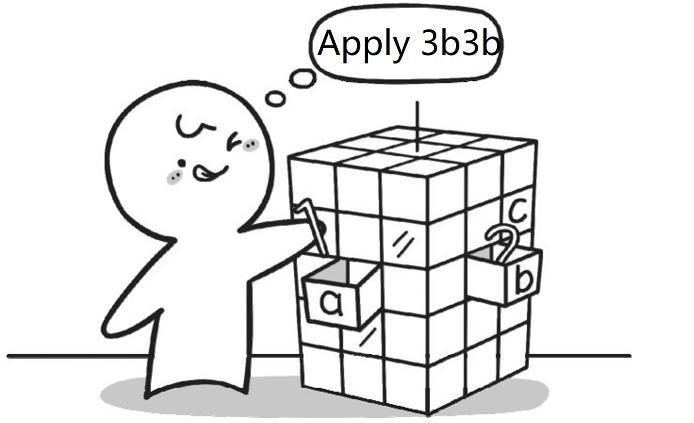The role of the “=” assignment symbol is the equivalent of a hand, put Numbers in the small house.

``````int a, b, c;
``````

This sentence is in the “skyscraper” of the computer application for three small house called a, b and c respectively. (note: there is a space between the int and a, a, b and c respectively use commas between, a semicolon at the end of the end).

Next, we make a little house and the house b to record two Numbers 1 and 2 respectively, specific as follows:

``````a = 1;
b = 2;
``````

Description: here there is a “=”, this is not equal to the number, it is called the number given (also known as assignment), similar to an arrow “please”, meaning is assigned to the content of the “=” the right “=” to the left.For example, the number 1 for a small house, so that the computer will know that a small house in a store is the number 1.

Then, the small house a and b together in the small house, and then put the result in a small house in the c.

``````c = a + b;
``````

The Computer will execute this formula is divided into two steps: the first step to calculate a + b, the second step again to assign the value of a + b “=” to the right of the c.

At this point, it’s almost finished, we sum up:

``````int a, b, c;
a = 1;
b = 2;
c = a + b;
``````

Many students think, now already all finished?You forget the most important step, don’t rush to look down, think about forget?

Ah!Did you forget your answers output.

Think if mom ask you how much one plus two, you say: “I calculate, but they don’t want to tell you!”, estimates that will get a hit, don’t!

Let us recall, how to output the results.

Yes, use printf statements.That how to store small building c the number of output?According to section 2 of this chapter to learn knowledge, as long as put to output the contents of the double quotation marks inside is ok, the code is as follows:

``````printf (" c ");
``````

Guess what computer output at this time?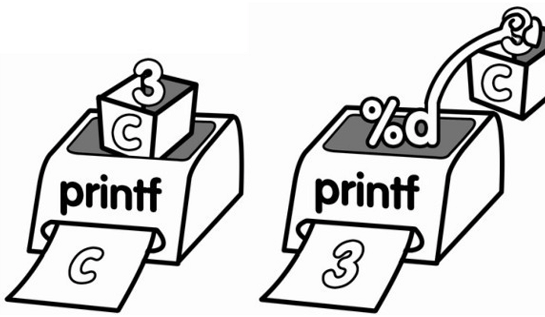Yes, relentlessly to output a c.

So how to output the value of c?

At this time we want to make another role played. “%d” “%d” is actually a “debt collection”, or it can be said to be “beggar”.Its full-time job is “money” to others!Then how should we use it?

``````    printf("%d", c);
``````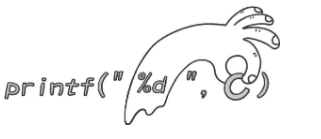Put “% d” between double quotes, put the small house c after the double quotation marks, and use commas. The printf found inside double quotation marks a “debt collection”, will know that at this point need to output a specific value, rather than sign, double quotes to the back of the little house c for the exact value.

Ok, then add the C language code framework, computer to add operation complete code is as follows:

``````#include <stdio.h>
#include <stdlib.h>
int main()
{
int a, b, c;
a=1;
b=2;
c=a+b;
printf("%d", c);
system("pause");
return 0;
}
``````

Now go to give it a try.

## The bedroom for digital - variables

In last section, we learned that computers use to remember one little house Numbers.Computer has many different kinds of small house.

``````  int a;
``````

Represents the apply to the computer for a small house, used to store values, name is a small house.Int and a there is a space between, at the end of a has a semicolon, said the end of the sentence.

If you want to apply for multiple small house, behind a continue with b and c, separated by commas.Such as:

`````` int a, b, c;
``````

There’s a small problem, is to apply for the “little house” name.In principle can literally: can be separate letters, such as a, b or x;Can be a combination of multiple letters, such as aaa, ABC or book;Also can be a combination of letters and Numbers, such as a1 or abc123.Of course, there are some restrictions, if you want to know, please see appendix A.

Here, there may be many students want to ask, just what is meant by the int?

Actually, int control used to store the number of types of small house, said you now apply for small house only integers.

The abbreviation of int is the English word integer (integer).

If you want to put a decimal?

We use a float to apply for a small house, used to store the decimal, form is as follows:

``````float a;
``````

In this way, the small house can be used to store a decimal, such as:

``````float a;
a=1.5;
printf("%f", a);
``````

Means to apply for a small house. A, used to store the decimal in the decimal 1.5.

Note: in C, the decimal is called a floating-point number, using float said.

Before using the printf statements output an integer, using the “% d”.At this time to output decimal, want to use “% f”.

Ok, let’s sum up, the “little house” in our C language terminology is called a variable.Int and float small house is used to store what type of number, we call it here “variable type” or “data types”.

Similar int a;Or float a;Form, we called the “define variables”, their syntax format is as follows:

[English] small house type the name of a small house, the name of a small house; “Term” variable of the type of the variable name, the name of the variable; [code] int a, b; We now know that int a;Said to apply for a small house used to store an integer a, which defines a variable a, to hold the integer.And float a;Said to apply for a small house used to store a decimal a, which defines a floating-point type (solid) variables to store a floating point number (decimal).

Another interesting question, look at the HTML code is as follows:

``````#include <stdio.h>
#include <stdlib.h>
int main()
{
int a;
a=1;
a=2;
printf("%d", a);

system("pause");
return 0;
}
``````

The computer after execution of the code above, please will output 1 or 2?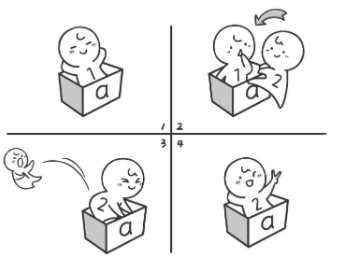## Data output - I say how is going well

In section 4 of this chapter, we have learned how to make the computer do add operation, but the computer in the output, only shows a result, such inhumane. If it outputs the entire arithmetic equation, for example: 1 + 2 = 3. How to write it?

The new code:

``````    #include <stdio.h>
#include <stdlib.h>
int main()
{
int a, b, c;
a=1;
b=2;
c=a+b;
printf("%d+%d=%d", a, b, c);

system("pause");
return 0;
}
``````

The original code：

``````    #include <stdio.h>
#include <stdlib.h>
int main()
{
int a, b, c;
a=1;
b=2;
c=a+b;
printf("%d", c);
system("pause");
return 0;
}
``````

Read the code carefully you will find that the new code and is not the same as the original code only a printf statements.Good, we now to analysing printf (” % d + % d = % d”, a, b, c);。

Printf statements will only output the inside of the double quotes, double quotes outside of the part is just within the double quotes on some added.

For example, the printf (” % d + % d = % d “, a, b, c);This line and the inside of the double quotation marks is % d + % d = % d, then the computer when I was in the output in strict accordance with the % d + % d = % d to execute, the output must be in the form of % d + % d = % d.

When a computer is when you meet the first “% d” know “debt collection” is coming, so it has to double quotation marks at the back of the variables, debt collection, the first of the list is a, then to a debt collection.A value is 1, the first is 1 “% d”.

The second is “+”, then output.

Third is “% d”, also to the back of the double quotation marks to debt collection, because at first a debt has been pleasing, therefore to row in the 2 b debt collection.The value of b is 2, then the “% d” is 2.

The fourth is the “=”, so the output.

Fifth or “% d”, also to the back of the double quotation marks to debt collection, because in the first of a and b in the second for a debt had been made, and therefore to row in the 3 c debt collection.A value of 3 c, so the “% d” finally get is 3.

The content of the final output is 1 + 2 = 3.

Please note that usually the number of inside double quotes “% d”, and behind the number of variables are equal, they are one to one correspondence.If there is no one-to-one correspondence, from the perspective of the C language grammar is not wrong, but the irrational, it is best to avoid such cases.

## - I said what is what for data input

We have learned how to do a totalizer, but current addition calculator is not enough human, when calculating the number of two and each time, all need to modify the C language code, and then recompile run to get results, obviously such a totalizer is not some people like to use, how to let the user to enter any two Numbers can directly get the results?

As we know, let the computer speak with printf, then lets the computer go to learn to listen to what?The scanf will tell you hear the content of the program.

Computer “speak” process, we called “output”, the computer process of “listening”, we are called the “read”.Ok, take a look at, the computer is how to read.

The scanf grammar is similar to the printf grammar, for example, we are going to read a number from keyboard, placed in a small house, the code is as follows: scanf("%d”, &a); You see, and the output of a small house statement printf (” % d “, a);Is same, only the following two different.

The first is: read in the word is to use the scanf, while the output is the use of the word printf.

The second place is: read more output in front of a a “&” symbol.

We called “&” symbol “address-of operator”, hereinafter referred to as “address”.Its role is to get the address of a small house.

The scanf (” % d “, & a);This sentence can be understood as: we want to transfer from external to internal computer a numeric value, and the need to store this value to the specified “Numbers for a” box.So we need to know a small box within the computer address.Just like the postman deliver letters, data to be transmitted is letters, small box is a box number.

That you may ask, why when reading to get the address of a small house, and the output is not need?When reading data, the computer needs to be read in the value of the store in a small house (that is, a variable), the need to know that you specify the address of the small house a, can put value accurately in among them;But in the output value has been in a small house, so you can direct the output to the screen.

Make an example: if you want to go to a classroom, so you need to know the address of the classroom before the class, so you can go to;But if the class was over, you need to get out of the classroom, because now you have in the classroom, so wouldn’t need the address of the classroom.

If you want to read in two Numbers from keyboard, respectively to the small house and the house b?Here are the following two kinds of writing.

The first:

The scanf (” % d “, & a); The scanf (” % d “, & b); The second:

The scanf (” % d % d “, & a & b); The second method is relatively simple, don’t need a space between the two “% d”, “& a” and “&” b “comma separated.

Read in two Numbers from keyboard, output the number of the two and the complete code is as follows:

``````    #include <stdio.h>
#include <stdlib.h>
int main()
{
int a, b, c;
scanf("%d%d", &a, &b);
c=a+b;
printf("%d+%d=%d", a, b, c);

system("pause");
return 0;
}
``````

Well, to summarize, in C printf is said “go out”, computer is computer need to tell you;And the scanf is “listen in” computer, you need to tell the computer.

Next, we want to make more human addition calculator - with a hint of reading, and output.

``````    #include <stdio.h>
#include <stdlib.h>
int main()
{
int a, b, c;
printf("This is an additive calculator, you are welcome to use\n");
printf("--------------------------------\n");
printf("Please enter the first number (please press enter after the input)\n");
scanf("%d", &a);
printf("Please enter the second number (please press enter after the input)\n");
scanf("%d", &b);
c=a+b;
printf("The sum of them is %d", c);

system("pause");
return 0;
}
``````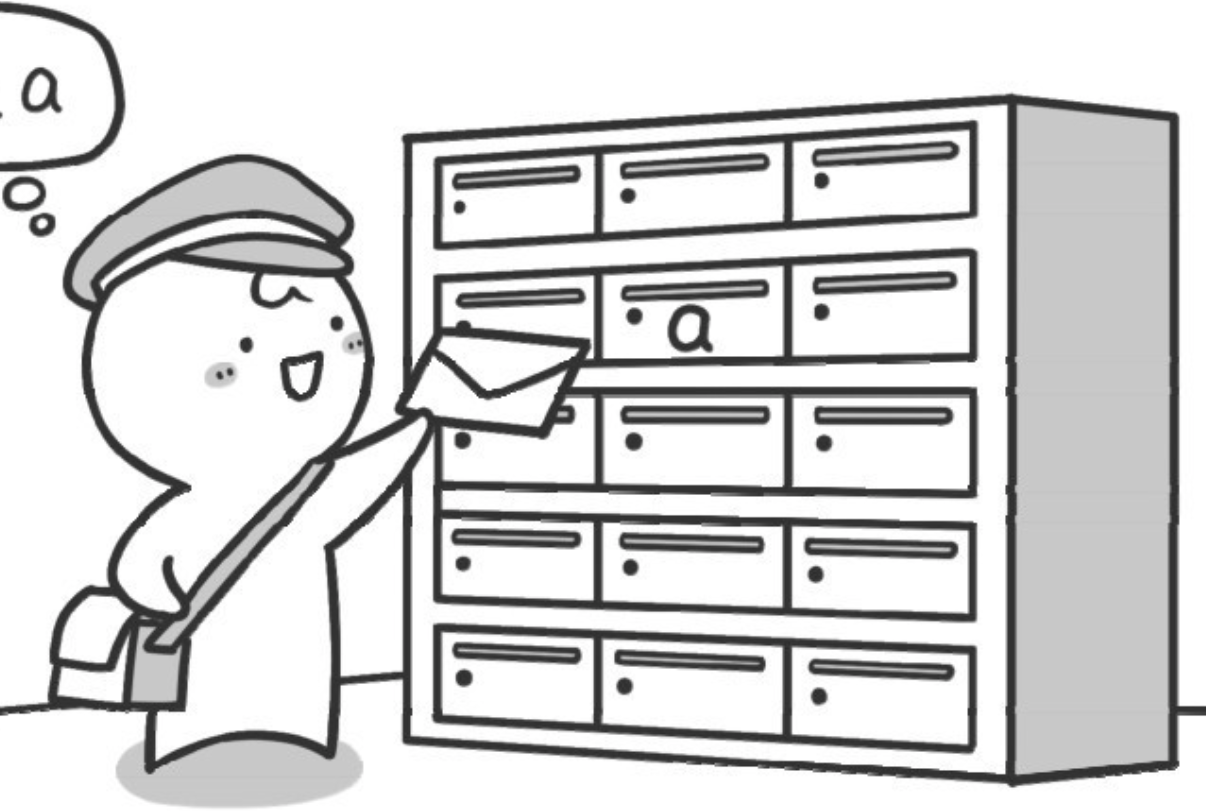## How many kinds of small houses are there

In previous sections, we have learned that if a computer wants to “remember” a value, it must apply for a small house in its brain “skyscraper”. For example:

``````    int  a, b, c ;
``````

That is to apply for three kinds of small houses called a, B and C. These three kinds of small houses can only be used to store integers (integer data).

``````    #include <stdio.h>
#include <stdlib.h>
int main()
{
float a;
a=3.1415926535897932;
printf("%.15f", a);

system("pause");
return 0;
}
``````

Code 2:

``````    #include <stdio.h>
#include <stdlib.h>
int main()
{
double a;
a=3.1415926535897932;
printf("%.15f", a);

system("pause");
return 0;
}
``````

Through observation, we find that there is only one difference between code 1 and code 2. In code 1, float is used to apply for small house a, while in code 2, double is used to apply for small house a. At the time of output, the placeholder used in printf in both pieces of code is “F”. The “. 15” between “%” and “F” in the code means 15 decimal places (rounded). In particular, the placeholder used when reading double type data with scanf is “LF” (note that this is not the number 1, but the letter L) instead of “F”. What’s up, you got the problem?! The output of code 1 after running is 3.141592740112573, which is obviously wrong from the 7th decimal place, while the output of code 2 is 3.141592653589793, which is completely correct. So we can find that double is more accurate than float. In addition, the range of numbers represented by float and double is also different. Please try it yourself.

``````    #include <stdio.h>
#include <stdlib.h>
int main()
{
char a;
scanf("%c", &a);
printf("The character you just entered is%c", a);

system("pause");
return 0;
}
``````

We enter a character X and press enter. The result is shown in Figure 2-18. Of course, you can try other characters.

Think about this code, if you input a string of letters instead of a letter, what will the computer output? I’m sorry! The computer will only output the first letter you type.

``````    #include <stdio.h>
#include <stdlib.h>
int main( )
{
char a;
scanf("%c", c);
printf("%d", c);
system("pause");
return 0;
}
``````
``````    #include <stdio.h>
#include <stdlib.h>
int main()
{
char a;
scanf("%c", &a);
printf("The following character is%c", a+1);

system("pause");
return 0;
}
``````

Think about it. Why is the character after a character plus 1?

## Clear the clouds and see the moon

Through the previous study, we have known that if the computer wants to “remember” a certain value, it must apply for a small house in the “skyscraper” of the computer brain. For example, we need to calculate the sum of any two numbers

``````    #include <stdio.h>
#include <stdlib.h>
int main()
{
int a, b, c;
scanf("%d%d", &a, &b) ;
c=a+b;
printf("%d+%d=%d", a, b, c);

system("pause");
return 0;
}
``````

In fact, this small house C is redundant and can be written directly as follows:

``````    #include <stdio.h>
#include <stdlib.h>
int main()
{
int a, b;
scanf("%d%d", &a, &b);
printf("%d+%d=%d", a, b, a+b);

system("pause");
return 0;
}
``````

Of course, if you only want to calculate the value of 4 + 5, it can be simpler:

``````    #include <stdio.h>
#include <stdlib.h>
int main()
{
printf("%d",4+5);
system("pause");
return 0;
}
``````

## Report problems to us

Hi, if you find something need improved. Please feel free to contact us feedback. We will buy you a coffee if your advise is adopted. Thx.